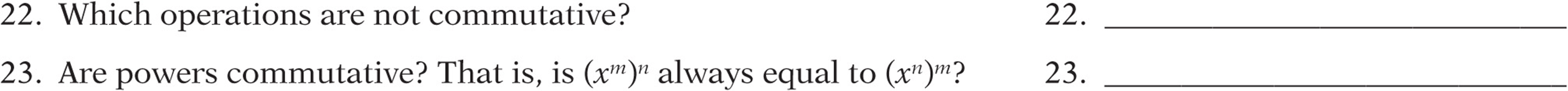﻿ ﻿Laws of Arithmetic - ESSENTIAL PRE-ALGEBRA SKILLS - SAT Test Prep

## CHAPTER 7ESSENTIAL PRE-ALGEBRA SKILLS

### Lesson 2: Laws of Arithmetic

The Laws of Arithmetic

When evaluating expressions, you don”t always have to follow the order of operations strictly. Sometimes you can play around with the expression first. You can commute (with addition or multiplication), associate (with addition or multiplication), or distribute (multiplication or division over addition or subtraction). Know your options!

When simplifying an expression, consider whether the laws of arithmetic help to make it easier.

Example:

57(71) + 57(29) is much easier to simplify if, rather than using the order of operations, you use the “distributive law” and think of it as.

The Commutative and
Associative Laws

Whenever you add or multiply terms, the order of the terms doesn”t matter, so pick a convenient arrangement. To commute means to move around. (Just think about what commuters do!)

Example:(Think about why the second arrangement is more convenient than the first!)

Whenever you add or multiply, the grouping of the terms doesn”t matter, so pick a convenient grouping. To associate means to group together. (Just think about what an association is!)

Example:(Why is the second grouping more convenient than the first?)

Whenever you subtract or divide, the grouping of the terms does matter. Subtraction and division are neither commutative nor associative.

Example:(So you can”t “commute” the numbers in a difference until you convert it to addition:.)(So you can”t “commute” the numbers in a quotient until you convert it to multiplication:.)

The Distributive Law

When a grouped sum or difference is multiplied or divided by something, you can do the multiplication or division first (instead of doing what”s inside parentheses, as the order of operations says) as long as you “distribute.” Test these equations by plugging in numbers to see how they work:

Example:Distribution is never something that you have to do. Think of it as a tool, rather than a requirement. Use it when it simplifies your task. For instance, 13(832 + 168) is actually much easier to do if you don”t distribute:. Notice how annoying it would be if you distributed.

Use the distributive law “backwards” whenever you factor polynomials, add fractions, or combine “like” terms.

Example:Follow the rules when you distribute! Avoid these common mistakes:

Example:is not(Tempting, isn”t it? Check it and see!)is notConcept Review 2: Laws of Arithmetic

Simplify the following expressions, and indicate what law(s) of arithmetic you use to do it. Write D for distribution, CA for commutative law of addition, CM for commutative law of multiplication, AA for associative law of addition, and AM for associative law of multiplication.Look carefully at the following equations. If the equation is always true, write the law of arithmetic that justifies it (D, CA, CM, AA, or AM). If it is false, rewrite the right side of the equation to make it true.Rewrite the expressionaccording to the following laws:

11. distributive law: ____________________

12. commutative law of addition: ____________________

13. commutative law of multiplication: ____________________

Do the following calculations mentally (no calculator!) by using the appropriate laws of arithmetic:If a and b are not 0:21. The distributive law says that only ____________________ or ____________________ can be distributed over grouped __________ or __________.SAT Practice 2: Laws of Arithmetic

1. The difference of two integers is 4 and their sum is 14. What is their product?

(A) 18

(B) 24

(C) 36

(D) 45

(E) 56

2. For all real numbers x and y,3. If, then(A) 120

(B) 200

(C) 280

(D) 570

(E) 578

4. The expressionis equivalent to which of the following expressions?(A) none

(B) II only

(C) I and II only

(D) I and III only

(E) I, II, and III

5. If, what is the value of y?

(A) –2

(B) –1

(C) 0

(D) 1

(E) 3

6. For all real numbers x,(A) x7. If,, and, then which of the following expresses the correct ordering of a, b, and c?8. Which of the following is equivalent tofor all x?(D) 145x

(E) 25x2

9. Which of the following statements must be true for all values of x, y, and z?(A) I only

(B) I and II only

(C) I and III only

(D) I and III only

(E) I, II, and III

10. The symbolrepresents one of the fundamental arithmetic operators: +, –, ×, or ÷. Iffor all positive values of x and y, thencan represent

(A) + only

(B) × only

(C) + or × only

(D) – only

(E) ÷ only

Answer Key 2: Laws of Arithmetic

Concept Review 2

1.(commutative law of arithmetic)

2.(distributive law)

3. 10m3n4 (commutative law of multiplication and associative law of multiplication)

4.(associative law of arithmetic and commutative law of arithmetic)

5. false:(Find a common denominator, then add numerators.)

6. true: distributive law

7. false:(Don”t ever distribute a power over addition. Expand and “FOIL” (First + Outside + Inside + Last).)

8. false: 15c2x (Don”t ever distribute multiplication over multiplication. Use the associative and commutative laws of multiplication.)

9. false: 3(2)6 + 35 (You can distribute, but don”t multiply before doing the powers!)

10. true: distributive law (Remember you can distribute division over addition!)14. 300 (Reorder:15. 3,000y4 (Reorder:16. 19,000 (Use the distributive law.)

17. 10,000x2 (You can”t “FOIL” here! Reorder: (4 × 25)18. They”re reciprocals. (Their product is 1.)

19. They”re the same.

20. They”re opposites. (Their sum is 0.)

21. multiplication or division over addition or subtraction

22. subtraction and division

23. yes

SAT Practice 23. B Don”t solve for x. It”s too hard, and it”s unnecessary.7. A Each number has 100 factors, to make them simpler to compare: a = 60(99)99 = 30(99)99 = 90(99)999. C Just remember that only addition and multiplication are commutative and associative. If you”re not convinced, you might plug in 1, 2, and 3 for x, y, and z, and notice that equation II is not true.﻿